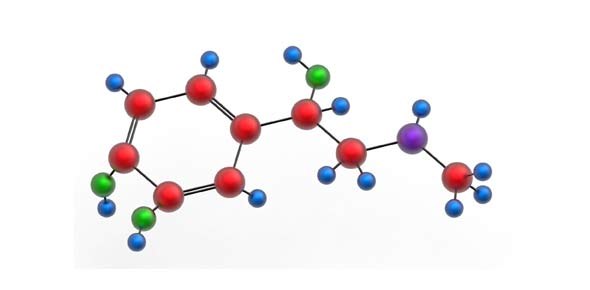# Multivalent Compounds (Cilts)

Approved & Edited by ProProfs Editorial Team
At ProProfs Quizzes, our dedicated in-house team of experts takes pride in their work. With a sharp eye for detail, they meticulously review each quiz. This ensures that every quiz, taken by over 100 million users, meets our standards of accuracy, clarity, and engagement.
| Written by Zach88
Z
Zach88
Community Contributor
Total Contribution - 3 | Total attempts - 4,215
Questions: 10 | Attempts: 2,238SettingsTry these practice questions!

• 1.

### What is the formula for Tin (II) Oxide?

Explanation
The formula for Tin (II) Oxide is SnO.

Rate this question:

• 2.

### What is the formula for Copper (II) Oxide?

Explanation
The formula for Copper (II) Oxide is CuO. This is because Copper (II) refers to the copper ion with a charge of +2, and Oxide refers to the oxygen ion with a charge of -2. To balance the charges, one copper ion combines with one oxygen ion, resulting in the formula CuO.

Rate this question:

• 3.

### What is the correct subscript for the following formula Iron (II) Carbonate = FeCO_

• A.

1

• B.

2

• C.

3

• D.

4

C. 3
Explanation
The correct subscript for the formula Iron (II) Carbonate is 3. This is because the formula FeCO3 indicates that there are three oxygen atoms present in the compound.

Rate this question:

• 4.

### What is the correct name for the formula SnSO4?

• A.

Tin (III) Sulfate

• B.

Tin (IV) Sulfide

• C.

Tin (II) Sulfate

• D.

Tin (I) Sulfur

C. Tin (II) Sulfate
Explanation
The correct name for the formula SnSO4 is Tin (II) Sulfate. This is because the Sn in the formula represents tin, which has a +2 charge in this compound. The SO4 represents sulfate, which has a -2 charge. In order to balance the charges, there needs to be two tin ions to every one sulfate ion, resulting in the name Tin (II) Sulfate.

Rate this question:

• 5.

### What is the correct name for the formula for Pb2(SO4)4?

Explanation
The correct name for the formula Pb2(SO4)4 is Lead (IV) Sulfate. In this compound, lead has a charge of 4+ and sulfate has a charge of 2-. The Roman numeral IV indicates the charge of lead, and the name "sulfate" represents the polyatomic ion SO4. By combining these names, we get the correct name for the compound, Lead (IV) Sulfate.

Rate this question:

• 6.

### What is the correct name for the formula FeI3?

• A.

Iron (III) Iodide

• B.

Iron (II) Iodine

• C.

• D.

Iron (III) Iodine

A. Iron (III) Iodide
Explanation
The correct name for the formula FeI3 is Iron (III) Iodide. This is because the formula indicates that there are three iodide ions (I-) for each iron ion (Fe3+). The Roman numeral III in parentheses indicates the charge of the iron ion, which is necessary to balance the charges of the ions in the compound. The other options, Iron (II) Iodine, Lead (IV) Iodide, and Iron (III) Iodine, do not correctly represent the composition and charge of the compound.

Rate this question:

• 7.

### What is the correct name for Fe(NO3)3?

• A.

Iron (III) Nitrate

• B.

Iron (II) Nitrate

• C.

Iron (I) Nitrate

• D.

Iron (IV) Nitrate

A. Iron (III) Nitrate
Explanation
Fe(NO3)3 is correctly named Iron (III) Nitrate. This is because the compound contains iron (Fe) in its +3 oxidation state, which is indicated by the Roman numeral III in the name. Nitrate (NO3) is the anion present in the compound.

Rate this question:

• 8.

### What is the correct name for Cu2SO4?

• A.

Copper (I) Silicate

• B.

Copper (I) Sulfite

• C.

Copper (I) Sulfide

• D.

Copper (I) Sulfate

D. Copper (I) Sulfate
Explanation
The correct name for Cu2SO4 is Copper (I) Sulfate. This is because the compound Cu2SO4 consists of copper ions with a +1 charge (Cu+), and sulfate ions with a -2 charge (SO4-). When naming ionic compounds, the Roman numeral is used to indicate the charge of the cation. In this case, the Roman numeral (I) indicates that the copper ion has a +1 charge. The anion, which is the sulfate ion, does not require a Roman numeral because it is a polyatomic ion with a fixed charge. Therefore, the correct name for Cu2SO4 is Copper (I) Sulfate.

Rate this question:

• 9.

### What is the correct name for Cu2CO3?

• A.

Copper (II) Carbonate

• B.

Copper (III) Carbonate

• C.

Copper (I) Carbonate

• D.

Copper (II) Bicarbonate

C. Copper (I) Carbonate
Explanation
Copper (I) Carbonate is the correct name for Cu2CO3. In this compound, copper has a +1 oxidation state, indicated by the Roman numeral I in parentheses. Carbonate is a polyatomic ion with a -2 charge. Therefore, the compound is formed by combining two copper ions with one carbonate ion, resulting in Cu2CO3.

Rate this question:

• 10.

### The formula for Lead (IV) Oxide is PbO

• A.

True

• B.

FalseBack to top